# Category Archives: Help Kids Learn Blog

Help kids learn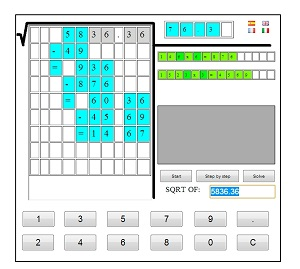# Calculate square root mentally

Calculate square root mentally

Until today I did not know Alexis Lemaire , the human calculator, but I read an article about it that surprised me so much that I have thought about putting some tricks to get calculate the square roots of head, ie mentally.
What Alexis has no name, at least to me it seems so impossible to get it and I can not imagine how has been able to calculate the thirteenth root of a 100 digit number!!! in only 3.6 seconds and the last time in the London Science Museum, calculating the thirteenth root of a 200 digit number, mentally of course, in less of 2 minutes to correctly give the result of 2,407,899,883,032,220.
But back to the techniques we use to find the square root of the head of a number.
1.- The first and most effective will be remember perfect squares, technique used by Alexis to its challenges. Obviously we will not be able to memorize so many numbers as he does, but we can start with the first and those simple as multiples of 10.
2.- Imagine now that I have memorized a few squares, for example the square of 20 is 400, but I need to calculate the square root of 484, which is near but how can I get exactly which is your square root? . So we can make use of 2 well-known formulas:$(x+1)^2 = x^2 + 2x + 1$
and$(x-1)^2 = x^2 - 2x + 1$
Mentally we know that the square of 21 is equal to the square of (20 +1 ) and according to the formula that we have seen corresponds to :$(20+1)^2 = 20^2 + 2*20 + 1 = 400 + 40 + 1 = 441$.
If we retain in head this number ( 441) we can still compute in the same way the next perfect square using the same formula:$(21+1)^2 = 21^2 + 2*21 + 1 = 441 + 42 + 1 = 484$.
Number that corresponds to the one we were looking for. Thus the square root of 484 is 22 .
3.- One last trick , if we need to calculate the square root of 16641 and it seems very large, we remove the last two digits , we are left with 166 , which is between 144 and 169 , as 169 is closer than 144 we’ll use this. The root of 169 is 13, 130 and 130 is 16900 We’re close , but as we have gone we ‘ll subtracting 130-1 apply the same formula we’ve seen. :$(130-1)^2 = 130^2 - 2*130 + 1 = 16900 - 260 + 1 = 16641$.
And there it is, our square root was 129.
Obviously not intended to reach the level of Alexis Lemaire , but perhaps this is sufficient to what we find ourselves in daily life .# Calculator Square Root on Facebook

Calculator Square Root is already on facebook, and hopes you share it with your friends so that this utility can be used by more people. Our first goal is to get 50 friends on Facebook to continue including other utilities that you recommend us.
Meanwhile we hope your suggestions of other tools that can be used in the education of our children, any similar utility for divisions?.
Also, you can leave comments for improvement of this utility, opinions and doubts about this tool. Did it run fast or the site was very slow?

# Square root exercises

The best way to learn to do something is by practicing exercises of that matter resolved. We offer you this application to do that.

With this utility you will have all exercises of square root solved that you want, because you are who put the exercise and the application will solve it step by step.
In this way you can set the example and check that you have correctly solved the exercise seeing the solution in the screen.
Do not forget that another good way to learn and practice the square root is to put your exercise and checking the result yourself calculating the square of the result and adding the rest resulting thus gain speed in your addition and multiplication as well.

# Square root of 2

We know how we can calculate by hand the square root, and we also know that the result of the square root of a number X is other number whose square is X. Then, the square root of 2 is the real number whose square is 2.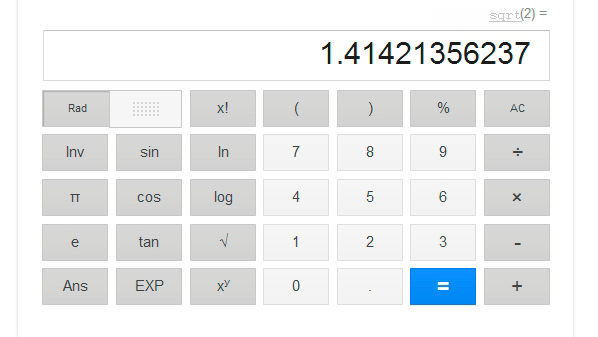The importance of this issue comes when we apply the Pythagorean Theorem to a square of size 1 by 1. The value of the hypotenuse is determined by the formula:$h^2=1^2+1^2$

That is:$h=\sqrt{2}$

This makes the square root of 2, possibly the first irrational number employee in the history of mathematics, remember that an irrational number is any real number that can not be expressed as a fraction m / n.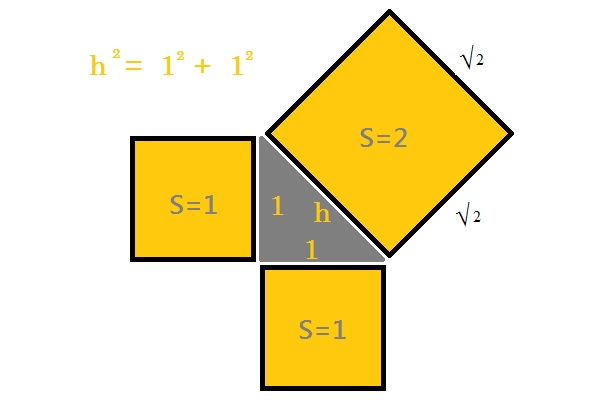As curious to note from wikipedia, without going into concepts that are beyond the middle school level discussed in this blog, is that its value has been calculated up to the incredible number of 137 438 953 444 decimal places by Yasumasa Kanada’s team in 1997, but, In February 2006 this record was eclipsed with the use of a home computer (200,000,000,000 decimal places). This makes him the second number, after π, where more decimals has been calculated.$\sqrt{2}=1,4142135623730950488016887242097\dots$

# What is the square root of a number?

The square root of a number is the inverse operation of the square of that number. That means:$\boxed{square (n) = n^2}$$\boxed{square root (n^2) = n}$

For example:$\boxed{square(7) = 7^2 = 49}$$\boxed{square root(49) = \sqrt{49} = 7}$

Then, the square root of a number is a value, that, multiplied by itself, gives the number.

# Calculate faster than a calculator

Trick to multiply faster than a calculator.

If you have 2 numbers of two digits, where the first digits is the same in both numbers, then you can solve the multiply in this way:$x = a \times 10 + b$$y = a \times 10 + c$ where$b + c = 10$

Then:$x \times y = [(a+1) \times a ] \bigcup [b \times c]$

For Example:$64 \times 66 = (7 \times 6) \bigcup (6 \times 4) = 4224$$51 \times 59 = (6 \times 5) \bigcup (1 \times 9) = 3009$$83 \times 87 = (9 \times 8) \bigcup (3 \times 7) = 7221$

and so on:$124 \times 126 = (13 \times 12) \bigcup (6 \times 4) = 15624$$114 \times 116 = (12 \times 11) \bigcup (6 \times 4) = 13224$

If you have questions you can see this video:

# Why is the sky blue?

The light from the Sun looks white. But it is really made up of all the colors of the rainbow. We can see the different colors of the spectrum by splitting the light with a prism. The spectrum is also visible when you see a rainbow in the sky. A prism is a specially shaped crystal. When white light shines through a prism, the light is separated into all its colors.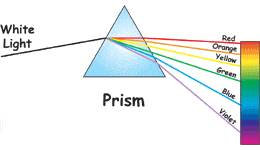The reason the sky is blue has to do with a concept called Rayleigh Scattering. Let’s say we have a gas, and we shine light on it. The light will hit the gas molecules, and get absorbed. Then, the gas molecules will emit the light back out at the same wavelength (color!), but in a new direction. Interestingly enough, blue light (shorter wavelength) gets absorbed more than red light (longer wavelength). This scattering of incoming light is Rayleigh Scattering!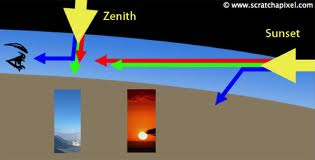Have you figured it out yet? Now imagine the sun, shining light towards the Earth. Remember, this is white light, which means it contains light of all visible colors at once! As the white light from the Sun hits the Earth’s atmosphere (a gas!), the molecules absorb and emit the blue light, and scatter it all around. So when you look up at the sky, you’re seeing all of this scattered blue light. But why is the sky paler near the horizon? At the horizon, light has to pass through more of the atmosphere, and in the same way blue light is scattered towards you, it ends up getting scattered away again, and so the other wavelengths are the ones that get through, since they are less affected by scattering.